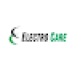News Ticker

## Unit, kWh, Wattage, Consumption, etc.

Friends, We all have an electricity connection at our home.  And If you see your electricity bill you must come across these terms as Unit, kWh, Wattage, Consumption. What is one unit of electricity? What is the cost of 1 unit of electricity? etc… So here I will explain all the terminology in very simple language and I hope after this article you will have a very clear cut picture about all these in your mind. First, we will understand what a unit of electricity is and how much is 1 unit of electricity.

## What is a Unit of Electricity?

A unit of electricity is a terminology to define a certain amount of electricity used or generated. For example, If you are using electricity at your home, then to charge you the bill for that it is necessary to measure how much electricity you have consumed. And it is measured in units which is equivalent to 1kWh. That is

1 Unit of electricity= 1kWh

Don’t worry we will discuss it in a detail.

If we expand the formula of 1 unit of electricity it will be 1 kiloWatt-hour. Here kilo means 1000. that is, you say it is 1000 Watt-hour. It means if anyone uses 1000 watt appliance for 1 hour then the electricity consumption will be 1 Unit which is 1kWh.

On the basis of the above definition, If you want to calculate the unit consumption of any appliance the formula will be

Unit consumed = (Wattage of appliance x Usage time in the hour)/1000 Unit or kWh

For example, if you have a 100-watt light bulb and you use it for 10 hours, then the

Unit consumed = (100 x 10)/1000 Unit or kWh

= 1 Unit or kWh

Similarly, if you have a 1,000-watt microwave oven that is used for 30 minutes, it will use 0.5 units of electricity, because 1,000 watts x (30 minutes / 60 minutes) = 500 watts-hours, which is equal to 0.5 kWh.

Another example, If we use a 60-watt fan for 20 hours it will consume 1200 watts-hours which is 1.2 kWh or 1.2 units.

## What is Power Consumption in kWh?

Now from the above discussion, you have already understood what is power consumption in kWh. Anyway, Power consumption is also the unit consumed by any appliance.

Power consumption in kWh is important because it is used to calculate the cost of operating a device or appliance. By understanding the power consumption of your appliances and devices, you can make informed decisions about how to use them more efficiently and reduce your energy costs.

Here are some examples of common appliances and their average power consumption in kWh

• The electricity consumption of a 5-star AC (1.5 tons) is approximately 1.5 units per hour, whereas a 3-star AC (1.5 tons) consumes 1.6 units every hour.
• Refrigerator: approximately 1.2 kWh per day
• Dishwasher: approximately 1.1 kWh per load
• Clothes washer: approximately 1.3 kWh per load
• Television: approximately 0.1 kWh per hour

## What is Wattage, Watt, or kW?

Wattage is the measure of the power of an appliance. Simply you can say that appliances having higher wattage will draw more power and consume more power or consume power at a higher rate.

You might have heard amount LED bulbs of wattage 9Watt, 12 Watt, or 20Watt. It simply indicates that the LED bulb has a wattage of 20 watts and is more powerful than 12 watt LED bulb. and  12 Watt LED bulb is more powerful than a 9-watt bulb.

in a similar way, you can also say that 20 watts LED bulb will consume power or electricity at a faster rate than a 12-watt bulb, and 12 watts LED bulb will consume power at a faster rate than 9 watt LED bulb.

## Summary:

If we conclude,

Unit is the terminology to indicate the amount of electricity used or generated, which is equivalent to 1kWh. Power consumption is the amount of electricity that a device or appliance uses over a period of time and wattage is the rating of an appliance showing its capacity.

I hope the article is helpful and easy to understand. Please comment if you have any doubts or queries. You may watch this video

1. ##### What is a Unit of Electricity?

A unit of electricity is a terminology to define a certain amount of electricity used or generated. For example, If you are using electricity at your home, then to charge you the bill for that it is necessary to measure how much electricity you have consumed. And it is measured in units which is equivalent to 1kWh. That is
1 Unit of electricity= 1kWhI am an Electrical Engineer and currently working as an Assistant Electrical Engineer in a distribution company. I am working with a distribution company for more than nine years. So here, I try to share my experience and knowledge to solve real-life problems related to electricity and electricity bills. More Posts

#### 4 Comments on What is 1 Unit of Electricity, Power Consumption kWh and Wattage kW?

1.Raghu // February 21, 2023 at 5:29 pm // Reply

Thank you, it was very informative article

•Amit Kumar // February 21, 2023 at 11:31 pm // Reply

Thanks..

2.Mr Joel // March 4, 2023 at 11:47 am // Reply

Hii Thanks for the Article, Very Informative Article. Waiting for the Next Article.

3.Electrocare // June 8, 2023 at 10:46 am // Reply

Thank you for sharing valuable information.